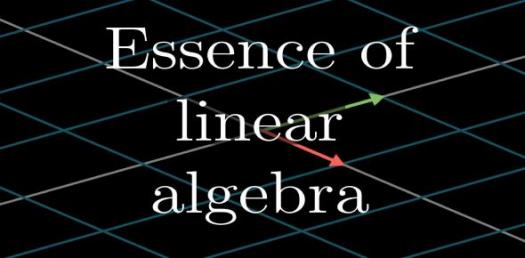# Linear Algebra Trivia Quiz

10 Questions | Total Attempts: 548SettingsLinear algebra is a subject of mathematics that involves linear expression or equation from a given linear function. Linear algebra is pivotal to almost all areas of mathematics. What else do you know about this branch of mathematics? Try this quiz

• 1.
What differentiates a linear expression from a linear equation?
• A.

Number of variables

• B.

Power of the first variable

• C.

Equality sign

• D.

Number of signs

• 2.
What is the number attached to the alphabet called?
• A.

Resultant

• B.

Coefficient

• C.

Constant

• D.

Variable

• 3.
What are alphabets called in linear Algebra?
• A.

Variable

• B.

Constant

• C.

Coefficient

• D.

Resultant

• 4.
What term is given to the equation true for all values of the variable?
• A.

Constant

• B.

Polynomial

• C.

Cofficient

• D.

Identity

• 5.
What kind of graph does a quadratic function have?
• A.

Hyperbolic

• B.

Parabolic

• C.

Embolic

• D.

Hyper-embolic

• 6.
Which of these is not a stage of Algebra?
• A.

Rhetorical

• B.

Analytical

• C.

Syncopated

• D.

Symbolic

• 7.
How many stages of Algebra do we have?
• A.

1

• B.

2

• C.

3

• D.

4

• 8.
What was used as the first systematic way to solve Linear algebra?
• A.

Lieberman's law

• B.

Cofficient

• C.

Determinants

• D.

Matrix

• 9.
Who published the theory of extension?
• A.

Cramer

• B.

Gauss

• C.

Leibniz

• D.

Hermann Grassmann

• 10.
What is the Latin meaning of the word matrix?
• A.

Array

• B.

Womb

• C.

Block

• D.

Worm

Related TopicsBack to top